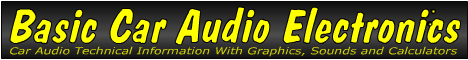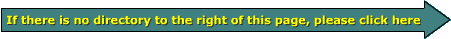Current

Current is the quantity of water, electrons... that flow past a given point in a given amount of time. The simplest way to understand flow is by looking at a river before and after a heavy period of rain. Before the rain, the flow of water in the river is moderate or slow. After the rain, there is much more current flowing. It's simple to understand that many more gallons of water are passing a given point in the river every second.

The diagram below shows a water pump, a couple of sections of pipe (marked 'A' & 'C') and a valve (marked 'B'). If the pressure (voltage) in pipe section 'A' is constant, and the valve is only slightly open the current flow into pipe 'C' is going to be relatively small. If we open the valve to its halfway point the restriction in the pipe has been reduced and the current flow will increase but will be less than the maximum possible flow. When the valve is fully opened, the flow will be as high as possible. As you can see, the flow of current is inversely proportional to the restriction presented by the valve. For electricity, resistance is the restriction in the electrical circuit.

small valve opening (high resistance) = reduced current flow
large valve opening (lower resistance) = increased current flow

Note:
You've no doubt heard the term 'high current amplifier'. On this site, you'll read about that and many more ways that the term 'high current' is used.

 You should remember: 1.Current is the rate of electron flow.

```

```